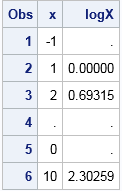I have previously discussed how to define functions that safely evaluate their arguments and return a missing value if the argument is not in the domain of the function. The canonical example is the LOG function, which is defined only for positive arguments. For example, to evaluate the LOG function on a sequence of (possibly non-positive) values, you can use the following IF-THEN/ELSE logic:

```data Log; input x @@; if x > 0 then logX = log(x); else logX = .; datalines; -1 1 2 . 0 10 ;   proc print; run;```On SAS discussion forums, I sometimes see questions from people who try to use the IFN function to accomplish the same logic. That is, in place of the IF-THEN/ELSE logic, they try to use the following one-liner in the DATA step:

`logX = ifn(x>0, log(x), .);`

Although this looks like the same logic, there is a subtle difference. All three arguments to the IFN function are evaluated BEFORE the function is called, and the results of the evaluation are then passed to the function. For example, if x= -1, then the SAS DATA step does the following:

1. Evaluate the Boolean expression x>0. When x= -1, the expression evaluates to 0.
2. Evaluate the second argument log(x). This happens regardless of the result of evaluating the first expression. When x= -1, the expression log(x) is invalid and the SAS log will report
NOTE: Invalid argument to function LOG
NOTE: Mathematical operations could not be performed at the following places. The results of the operations have been set to missing values.
3. Call the IFN function as logX = IFN(0, ., .), which results in assigning a missing value to logX.

In Step 2, SAS evaluates log(x) unconditionally for every value of x, which leads to out-of-domain errors when x is not positive. This is exactly the situation that the programmer was trying to avoid! In contrast, the IF-THEN/ELSE logic only evaluates log(x) when x is positive. Consequently, the SAS log is clean when you use the IF-THEN/ELSE statement.

There are plenty of situations for which the IFN function (and it's cousin, the IFC function) are useful, but for testing out-of-domain conditions, use IF-THEN/ELSE logic instead.

The post The IFN function versus the IF-THEN/ELSE statement in SAS appeared first on The DO Loop.